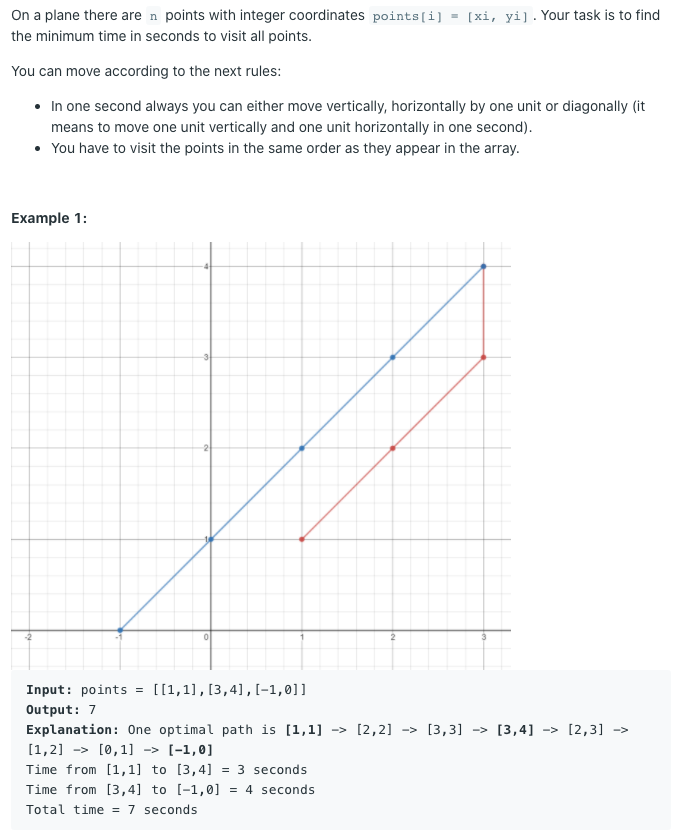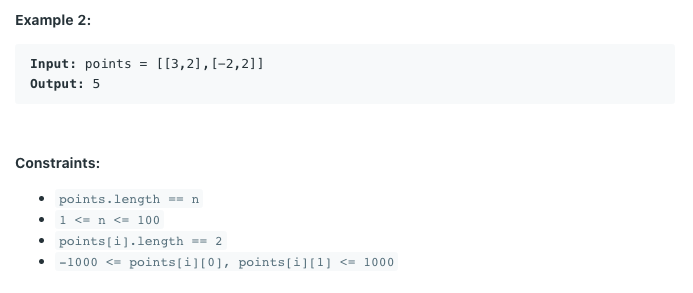# Leetcode 1266. Minimum Time Visiting All Points

0

## 알고리즘 문제풀이(Leetcode)

목록 보기
36/42class Solution:
def minTimeToVisitAllPoints(self, points: List[List[int]]) -> int:
# point <-- x
# point <-- y
# max(abs(x+1 - x), abs(y+1, y))
x = points
y = points
res = 0
for i, group in enumerate(points):
if i == 0: continue
x = abs(x - group)
y = abs(y - group)
res += max(x, y)
x = group
y = group

return res


https://leetcode.com/problems/minimum-time-visiting-all-points/